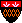Introduction to Automata Theory, Languages, and Computation

## Solutions for Chapter 5

Solutions for Section 5.1

Revised 11/11/01.

## Solutions for Section 5.1

### Exercise 5.1.1(a)

```     S -> 0S1 | 01
```

### Exercise 5.1.1(b)

```     S -> AB | CD
A -> aA | ε
B -> bBc | E | cD
C -> aCb | E | aA
D -> cD | ε
E -> bE | b
```

To understand how this grammar works, observe the following:

• A generates zero or more a's.
• D generates zero or more c's.
• E generates one or more b's.
• B first generates an equal number of b's and c's, then produces either one or more b's (via E) or one or more c's (via cD). That is, B generates strings in b*c* with an unequal number of b's and c's.
• Similarly, C generates unequal numbers of a's then b's.
• Thus, AB generates strings in a*b*c* with an unequal numbers of b's and c's, while CD generates strings in a*b*c* with an unequal number of a's and b's.

### Exercise 5.1.2(a)

Leftmost: S => A1B => 0A1B => 00A1B => 001B => 0010B => 00101B => 00101

Rightmost: S => A1B => A10B => A101B => A101 => 0A101 => 00A101 => 00101

### Exercise 5.1.5

```     S -> S+S | SS | S* | (S) | 0 | 1 | phi | e
```

The idea is that these productions for S allow any expression to be, respectively, the sum (union) of two expressions, the concatenation of two expressions, the star of an expression, a parenthesized expression, or one of the four basis cases of expressions: 0, 1, phi, and ε.

## Solutions for Section 5.2

### Exercise 5.2.1(a)

```                        S
/ | \
A  1  B
/ |   / |
0  A  0  B
/ |   / |
0  A  1  B
|     |
e     e
```

In the above tree, e stands for ε.

## Solutions for Section 5.3

### Exercise 5.3.2

```     B -> BB | (B) | [B] | ε
```

### Exercise 5.3.4(a)

Change production (5) to:

```     ListItem -> <LI> Doc </LI>
```

## Solutions for Section 5.4

### Exercise 5.4.1

Here are the parse trees:

```                  S                  S
/ |              / / | \
a  S             a S  b  S
/ | \ \           | \   |
a  S  b S          a  S  e
|    |             |
e    e             e
```

The two leftmost derivations are: S => aS => aaSbS => aabS => aab and S => aSbS => aaSbS => aabS => aab.

The two rightmost derivations are: S => aS => aaSbS => aaSb => aab and S => aSbS => aSb => aaSb => aab.

### Exercise 5.4.3

The idea is to introduce another nonterminal T that cannot generate an unbalanced a. That strategy corresponds to the usual rule in programming languages that an ``else'' is associated with the closest previous, unmatched ``then.'' Here, we force a b to match the previous unmatched a. The grammar:

```     S -> aS | aTbS | ε
T -> aTbT | ε
```

### Exercise 5.4.6

Alas, it is not. We need to have three nonterminals, corresponding to the three possible ``strengths'' of expressions:

1. A factor cannot be broken by any operator. These are the basis expressions, parenthesized expressions, and these expressions followed by one or more *'s.

2. A term can be broken only by a *. For example, consider 01, where the 0 and 1 are concatenated, but if we follow it by a *, it becomes 0(1*), and the concatenation has been ``broken'' by the *.

3. An expression can be broken by concatenation or *, but not by +. An example is the expression 0+1. Note that if we concatenate (say) 1 or follow by a *, we parse the expression 0+(11) or 0+(1*), and in either case the union has been broken.

The grammar:

```     E -> E+T | T
T -> TF | F
F -> F* | (E) | 0 | 1 | phi | e
```